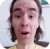The Spreadsheet::ParseExcel module can be used to read an Excel 95-2003 spreadsheet in Perl (files with the .xls extension).  The Spreadsheet::XLSX module is used to read an Excel 2007 spreadsheet in Perl (files with the .xlsx extension).

``use Spreadsheet::ParseExcel;``

These two lines are used to define the spreadsheet you are going to parse (example.xls in this example).

``````my \$ParseExcel = Spreadsheet::ParseExcel->new();
my \$ExcelFile = \$ParseExcel->Parse("/path/to/example.xls");``````

This markup will parse through each value in each cell in the spreadsheet.

``````foreach my \$worksheet ( \$workbook->worksheets() ) {

my ( \$first_row, \$last_row ) = \$worksheet->row_range();
my ( \$first_column, \$last_column ) = \$worksheet->col_range();

foreach my \$row ( \$first_row .. \$last_row ) {
foreach my \$column ( \$first_column .. \$last_column ) {

my \$cell = \$worksheet->get_cell( \$row, \$column );
next unless \$cell;

print "Row, Column = (\$row, \$column)\n";
print "Value       = ", \$cell->value(), "\n";
print "\n";
}
}
}``````

This will produce the following output like this:

``````Row, Column = (1,1)
Value       = Hello

Row, Column = (1,2)
Value       = World``````

We will never share your name or email with anyone. Enter your email if you would like to be notified when we respond to your comment.

Please enter 0bddc in the box below so that we can be sure you are a human.

Web design by yours truely - me, myself, and I   |   jeremy.canfield@freekb.net   |